The LOGISTIC Procedure
 PROC LOGISTIC Statement
PROC LOGISTIC <options> ;

The PROC LOGISTIC statement invokes the LOGISTIC procedure and optionally identifies input and output data sets, suppresses the display of results, and controls the ordering of the response levels. Table 51.1 summarizes the available options.

Table 51.1 PROC LOGISTIC Statement Options

Option

Description

Input/Output Data Set Options

displays estimated covariance matrix in OUTEST= data set

names the input SAS data set

specifies inital estimates SAS data set

specifies model information SAS data set

does not save covariance matrix in OUTMODEL= data set

specifies design matrix output SAS data set

outputs the design matrix only

specifies parameter estimates output SAS data set

specifies model output data set for scoring

Response and CLASS Variable Options

reverses sorting order of response variable

specifies maximum length of effect names

specifies sorting order of response variable

truncates class level names

Displayed Output Options

specifies significance level for confidence intervals

suppresses all displayed output

specifies options for plots

displays descriptive statistics

Large Data Set Option

does not copy input SAS data set for internal computations

Control of Other Statement Options

performs exact analysis only

specifies global options for EXACT statements

specifies global options for ROC statements

ALPHA=number

specifies the level of significance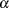for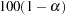% confidence intervals. The value number must be between 0 and 1; the default value is 0.05, which results in 95% intervals. This value is used as the default confidence level for limits computed by the following options:

Statement

Options

CONTRAST

EXACT

MODEL

ODDSRATIO

OUTPUT

PROC LOGISTIC

PLOTS=EFFECT(CLBAR CLBAND)

ROCCONTRAST

SCORE

You can override the default in most of these cases by specifying the ALPHA= option in the separate statements.

COVOUT

adds the estimated covariance matrix to the OUTEST= data set. For the COVOUT option to have an effect, the OUTEST= option must be specified. See the section OUTEST= Output Data Set for more information.

DATA=SAS-data-set

names the SAS data set containing the data to be analyzed. If you omit the DATA= option, the procedure uses the most recently created SAS data set. The INMODEL= option cannot be specified with this option.

DESCENDING
DESC

reverses the sorting order for the levels of the response variable. If both the DESCENDING and ORDER= options are specified, PROC LOGISTIC orders the levels according to the ORDER= option and then reverses that order. This option has the same effect as the response variable option DESCENDING in the MODEL statement. See the section Response Level Ordering for more detail.

EXACTONLY

requests only the exact analyses. The asymptotic analysis that PROC LOGISTIC usually performs is suppressed.

EXACTOPTIONS (options)

specifies options that apply to every EXACT statement in the program. The following options are available:

adds the observed sufficient statistic to the sampled exact distribution if the statistic was not sampled. This option has no effect unless the METHOD=NETWORKMC option is specified and the ESTIMATE option is specified in the EXACT statement. If the observed statistic has not been sampled, then the parameter estimate does not exist; by specifying this option, you can produce (biased) estimates.

BUILDSUBSETS

Some exact distributions are created by taking a subset of a previously generated exact distribution. When the METHOD=NETWORKMC option is invoked, this has the effect of using fewer than the desiredsamples; see the N= option for more details. The BUILDSUBSETS option suppresses this subsetting behavior and instead builds every distribution for sampling.

EPSILON=value

controls how the partial sums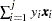are compared. value must be betweenand; by default, value=1E–8.

MAXTIME=seconds

specifies the maximum clock time (in seconds) that PROC LOGISTIC can use to calculate the exact distributions. If the limit is exceeded, the procedure halts all computations and prints a note to the LOG. The default maximum clock time is seven days.

METHOD=keyword

specifies which exact conditional algorithm to use for every EXACT statement specified. You can specify one of the following keywords:

DIRECT

invokes the multivariate shift algorithm of Hirji, Mehta, and Patel (1987). This method directly builds the exact distribution, but it can require an excessive amount of memory in its intermediate stages. METHOD=DIRECT is invoked by default when you are conditioning out at most the intercept, or when the LINK=GLOGIT option is specified in the MODEL statement.

NETWORK

invokes an algorithm described in Mehta, Patel, and Senchaudhuri (1992). This method builds a network for each parameter that you are conditioning out, combines the networks, then uses the multivariate shift algorithm to create the exact distribution. The NETWORK method can be faster and require less memory than the DIRECT method. The NETWORK method is invoked by default for most analyses.

NETWORKMC

invokes the hybrid network and Monte Carlo algorithm of Mehta, Patel, and Senchaudhuri (1992). This method creates a network, then samples from that network; this method does not reject any of the samples at the cost of using a large amount of memory to create the network. METHOD=NETWORKMC is most useful for producing parameter estimates for problems that are too large for the DIRECT and NETWORK methods to handle and for which asymptotic methods are invalid—for example, for sparse data on a large grid.

N=n

specifies the number of Monte Carlo samples to take when the METHOD=NETWORKMC option is specified. By default, n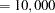. If the procedure cannot obtain n samples due to a lack of memory, then a note is printed in the SAS log (the number of valid samples is also reported in the listing) and the analysis continues.

Note that the number of samples used to produce any particular statistic might be smaller than n. For example, let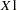and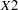be continuous variables, denote their joint distribution by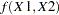, and let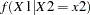denote the marginal distribution ofconditioned on the observed value of. If you request the JOINT test ofand, then n samples are used to generate the estimate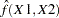of, from which the test is computed. However, the parameter estimate foris computed from the subset ofhaving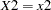, and this subset need not contain n samples. Similarly, the distribution for each level of a classification variable is created by extracting the appropriate subset from the joint distribution for the CLASS variable.

In some cases, the marginal sample size can be too small to admit accurate estimation of a particular statistic; a note is printed in the SAS log when a marginal sample size is less than 100. Increasing n will increase the number of samples used in a marginal distribution; however, if you want to control the sample size exactly, you can either specify the BUILDSUBSETS option or do both of the following:

• Remove the JOINT option from the EXACT statement.

• Create dummy variables in a DATA step to represent the levels of a CLASS variable, and specify them as independent variables in the MODEL statement.

ONDISK

uses disk space instead of random access memory to build the exact conditional distribution. Use this option to handle larger problems at the cost of slower processing.

SEED=seed

specifies the initial seed for the random number generator used to take the Monte Carlo samples when the METHOD=NETWORKMC option is specified. The value of the SEED= option must be an integer. If you do not specify a seed, or if you specify a value less than or equal to zero, then PROC LOGISTIC uses the time of day from the computer’s clock to generate an initial seed. The seed is displayed in the "Model Information" table.

STATUSN=number

prints a status line in the SAS log after every number Monte Carlo samples when the METHOD=NETWORKMC option is specified. The number of samples taken and the current exact p-value for testing the significance of the model are displayed. You can use this status line to track the progress of the computation of the exact conditional distributions.

STATUSTIME=seconds

specifies the time interval (in seconds) for printing a status line in the LOG. You can use this status line to track the progress of the computation of the exact conditional distributions. The time interval you specify is approximate; the actual time interval will vary. By default, no status reports are produced.

INEST=SAS-data-set

names the SAS data set that contains initial estimates for all the parameters in the model. If BY-group processing is used, it must be accommodated in setting up the INEST= data set. See the section INEST= Input Data Set for more information.

INMODEL=SAS-data-set

specifies the name of the SAS data set that contains the model information needed for scoring new data. This INMODEL= data set is the OUTMODEL= data set saved in a previous PROC LOGISTIC call. Note that the OUTMODEL= data set should not be modified before its use as an INMODEL= data set.

The DATA= option in the PROC LOGISTIC statement cannot be specified with this option; instead, specify the data sets to be scored in the SCORE statements. FORMAT statements are not allowed when the INMODEL= data set is specified; variables in the DATA= and PRIOR= data sets in the SCORE statement should be formatted within the data sets.

You can specify the BY statement provided that the INMODEL= data set is created under the same BY-group processing.

The CLASS, EXACT, MODEL, OUTPUT, ROC, ROCCONTRAST, TEST, and UNIT statements are not available with the INMODEL= option.

MULTIPASS

forces the procedure to reread the DATA= data set as needed rather than require its storage in memory or in a temporary file on disk. By default, the data set is cleaned up and stored in memory or in a temporary file. This option can be useful for large data sets. All exact analyses are ignored in the presence of the MULTIPASS option. If a STRATA statement is specified, then the data set must first be grouped or sorted by the strata variables.

NAMELEN=n

specifies the maximum length of effect names in tables and output data sets to be n characters, where n is a value between 20 and 200. The default length is 20 characters.

NOCOV

specifies that the covariance matrix not be saved in the OUTMODEL= data set. The covariance matrix is needed for computing the confidence intervals for the posterior probabilities in the OUT= data set in the SCORE statement. Specifying this option will reduce the size of the OUTMODEL= data set.

NOPRINT

suppresses all displayed output. Note that this option temporarily disables the Output Delivery System (ODS); see Chapter 20, Using the Output Delivery System, for more information.

ORDER=DATA | FORMATTED | FREQ | INTERNAL
RORDER=DATA | FORMATTED | INTERNAL

specifies the sorting order for the levels of the response variable. See the response variable option ORDER= in the MODEL statement for more information. For ordering of CLASS variable levels, see the ORDER= option in the CLASS statement.

OUTDESIGN=SAS-data-set

specifies the name of the data set that contains the design matrix for the model. The data set contains the same number of observations as the corresponding DATA= data set and includes the response variable (with the same format as in the DATA= data set), the FREQ variable, the WEIGHT variable, the OFFSET= variable, and the design variables for the covariates, including the Intercept variable of constant value 1 unless the NOINT option in the MODEL statement is specified.

OUTDESIGNONLY

suppresses the model fitting and creates only the OUTDESIGN= data set. This option is ignored if the OUTDESIGN= option is not specified.

OUTEST=SAS-data-set

creates an output SAS data set that contains the final parameter estimates and, optionally, their estimated covariances (see the preceding COVOUT option). The output data set also includes a variable named _LNLIKE_, which contains the log likelihood. See the section OUTEST= Output Data Set for more information.

OUTMODEL=SAS-data-set

specifies the name of the SAS data set that contains the information about the fitted model. This data set contains sufficient information to score new data without having to refit the model. It is solely used as the input to the INMODEL= option in a subsequent PROC LOGISTIC call. The OUTMODEL= option is not available with the STRATA statement. Information in this data set is stored in a very compact form, so you should not modify it manually.

PLOTS <(global-plot-options)><=plot-request<(options)>>
PLOTS <(global-plot-options)> =(plot-request<(options)><plot-request<(options)>>)

controls the plots produced through ODS Graphics. When you specify only one plot-request, you can omit the parentheses from around the plot-request. For example:

```   PLOTS = ALL
PLOTS = (ROC EFFECT INFLUENCE(UNPACK))
PLOTS(ONLY) = EFFECT(CLBAR SHOWOBS)
```

You must enable ODS Graphics before requesting plots. For example:

```   ods graphics on;
proc logistic plots=all;
model y=x;
run;
ods graphics off;
```

If the PLOTS option is not specified or is specified with no options, then graphics are produced by default in the following situations:

• If the INFLUENCE or IPLOTS option is specified in the MODEL statement, then the line-printer plots are suppressed and the INFLUENCE plots are produced.

• If you specify the OUTROC= option in the MODEL statement, then ROC curves are produced. If you also specify a SELECTION= method, then an overlaid plot of all the ROC curves for each step of the selection process is displayed.

• If the OUTROC= option is specified in a SCORE statement, then the ROC curve for the scored data set is displayed.

• If you specify ROC statements, then an overlaid plot of the ROC curves for the model (or the selected model if a SELECTION= method is specified) and for all the ROC statement models is displayed.

• If you specify the CLODDS= option in the MODEL statement, or specify an ODDSRATIO statement, then a plot of the odds ratios and their confidence limits is displayed.

For general information about ODS Graphics, see Chapter 21, Statistical Graphics Using ODS.

The following global-plot-options are available:

LABEL

displays the case number on diagnostic plots, to aid in identifying the outlying observations. This option enhances the plots produced by the DFBETAS, DPC, INFLUENCE, LEVERAGE, and PHAT options.

ONLY

suppresses the default plots. Only specifically requested plot-requests are displayed.

UNPACKPANELS | UNPACK

suppresses paneling. By default, multiple plots can appear in some output panels. Specify UNPACKPANEL to display each plot separately.

The following plot-requests are available:

ALL

produces all appropriate plots. You can specify other options with ALL. For example, to display all plots and unpack the DFBETAS plots you can specify plots=(all dfbetas(unpack)).

DFBETAS <(UNPACK)>

displays plots of DFBETAS versus the case (observation) number. This displays the statistics generated by the DFBETAS=_ALL_ option in the OUTPUT statement. The UNPACK option displays the plots separately. See Output 51.6.5 for an example of this plot.

DPC <(UNPACK)>

displays plots of DIFCHISQ and DIFDEV versus the predicted event probability, and colors the markers according to the value of the confidence interval displacement C. The UNPACK option displays the plots separately. See Output 51.6.8 for an example of this plot.

EFFECT<(effect-options)>

displays and enhances the effect plots for the model. For more information about effect plots and the available effect-options, see the section EFFECT Plots. See Outputs 51.2.11, 51.3.5, 51.4.8, 51.7.4, and 51.15.4 for examples of this plot.

INFLUENCE<(UNPACK)>

displays index plots of RESCHI, RESDEV, leverage, confidence interval displacements C and CBar, DIFCHISQ, and DIFDEV. These plots are produced by default when ods graphics on is specified. The UNPACK option displays the plots separately. See Outputs 51.6.3 and 51.6.4 for examples of this plot.

LEVERAGE<(UNPACK)>

displays plots of DIFCHISQ, DIFDEV, confidence interval displacement C, and the predicted probability versus the leverage. The UNPACK option displays the plots separately. See Output 51.6.7 for an example of this plot.

NONE

suppresses all plots.

ODDSRATIO<(oddsratio-options)>

displays and enhances the odds ratio plots for the model when the CLODDS= option or ODDSRATIO statements are also specified. For more information about odds ratio plots and the available oddsratio-options, see the section Odds Ratio Plots. See Outputs 51.7,51.2.9, 51.3.3, and 51.4.5 for examples of this plot.

PHAT<(UNPACK)>

displays plots of DIFCHISQ, DIFDEV, confidence interval displacement C, and leverage versus the predicted event probability. The UNPACK option displays the plots separately. See Output 51.6.6 for an example of this plot.

ROC<(ID=keyword)>

displays the ROC curve. If you also specify a SELECTION= method, then an overlaid plot of all the ROC curves for each step of the selection process is displayed. If you specify ROC statements, then an overlaid plot of the model (or the selected model if a SELECTION= method is specified) and the ROC statement models will be displayed. If the OUTROC= option is specified in a SCORE statement, then the ROC curve for the scored data set is displayed.

The ID= option labels certain points on the ROC curve. Typically, the labeled points are closest to the upper-left corner of the plot, and points directly below or to the right of a labeled point are suppressed. Specifying ID=PROB | CUTPOINT displays the predicted probability of those points, while ID=CASENUM | OBS displays the observation number. In case of ties, only the last observation number is displayed.

See Output 51.7.3 and Example 51.8 for examples of these ROC plots.

ROCOPTIONS (options)

specifies options that apply to every model specified in a ROC statement. The following options are available:

ALPHA=number

sets the significance level for creating confidence limits of the areas and the pairwise differences. The ALPHA= value specified in the PROC LOGISTIC statement is the default. If neither ALPHA= value is specified, then ALPHA=0.05 by default.

EPS=value

is an alias for the ROCEPS= option in the MODEL statement. This value is used to determine which predicted probabilities are equal. By default, EPS=1000*MACEPS (about 1E–12) for comparisons; however, EPS=0.0001 for computingfrom the "Association of Predicted Probabilities and Observed Responses" table when ROC statements are not specified.

ID=keyword-or-variable

displays labels on certain points on the individual ROC curves. This option is identical to, and overrides, the ID= suboption of the PLOTS=ROC option in the PROC statement. Specifying ID=PROB | CUTPOINT displays the predicted probability of an observation, while ID=CASENUM | OBS displays the observation number. In case of ties, the last observation number is displayed.

NODETAILS

suppresses the display of the model fitting information for the models specified in the ROC statements.

OUT=SAS-data-set-name

is an alias for the OUTROC= option in the MODEL statement.

WEIGHTED

uses frequencyweight in the ROC computations (Izrael et al.; 2002) instead of just frequency. Typically, weights are considered in the fit of the model only, and hence are accounted for in the parameter estimates. The "Association of Predicted Probabilities and Observed Responses" table uses frequency only, and is suppressed when ROC comparisons are performed.

SIMPLE

displays simple descriptive statistics (mean, standard deviation, minimum and maximum) for each continuous explanatory variable. For each CLASS variable involved in the modeling, the frequency counts of the classification levels are displayed. The SIMPLE option generates a breakdown of the simple descriptive statistics or frequency counts for the entire data set and also for individual response categories.

TRUNCATE

determines class levels by using no more than the first 16 characters of the formatted values of CLASS, response, and strata variables. When formatted values are longer than 16 characters, you can use this option to revert to the levels as determined in releases previous to SAS 9.0. This option invokes the same option in the CLASS statement.

### EFFECT Plots

Only one EFFECT plot is produced by default; you must specify other effect-options to produce multiple plots. For binary response models, the following plots are produced when an EFFECT option is specified with no effect-options:

• If you only have continuous covariates in the model, then a plot of the predicted probability versus the first continuous covariate fixing all other continuous covariates at their means is displayed. See Output 51.7.4 for an example with one continuous covariate.

• If you only have classification covariates in the model, then a plot of the predicted probability versus the first CLASS covariate at each level of the second CLASS covariate, if any, holding all other CLASS covariates at their reference levels is displayed.

• If you have CLASS and continuous covariates, then a plot of the predicted probability versus the first continuous covariate at up to 10 cross-classifications of the CLASS covariate levels, while fixing all other continuous covariates at their means and all other CLASS covariates at their reference levels, is displayed. For example, if your model has four binary covariates, there are 16 cross-classifications of the CLASS covariate levels. The plot displays the 8 cross-classifications of the levels of the first three covariates while the fourth covariate is fixed at its reference level.

For polytomous response models, similar plots are produced by default, except that the response levels are used in place of the CLASS covariate levels. Plots for polytomous response models involving OFFSET= variables with multiple values are not available.

See Outputs 51.2.11, 51.3.5, 51.4.8, 51.7.4, and 51.15.4 for examples of effect plots.

The following effect-options specify the type of graphic to produce.

AT(variable=value-list | ALL<...variable=value-list | ALL>)

specifies fixed values for a covariate. For continuous covariates, you can specify one or more numbers in the value-list. For classification covariates, you can specify one or more formatted levels of the covariate enclosed in single quotes (for example, A=’cat’ ’dog’), or you can specify the keyword ALL to select all levels of the classification variable. You can specify a variable at most once in the AT option. By default, continuous covariates are set to their means when they are not used on an axis, while classification covariates are set to their reference level when they are not used as an X=, SLICEBY=, or PLOTBY= effect. For example, for a model that includes a classification variable A={cat,dog} and a continuous covariate X, specifying AT(A=’cat’ X=7 9) will set A to cat when A does not appear in the plot. When X does not define an axis it first produces plots setting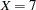and then produces plots setting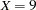. Note in this example that specifying AT( A=ALL ) is the same as specifying the PLOTBY=A option.

FITOBSONLY

computes the predicted values only at the observed data. If the FITOBSONLY option is omitted and the X-axis variable is continuous, the predicted values are computed at a grid of points extending slightly beyond the range of the data (see the EXTEND= option for more information). If the FITOBSONLY option is omitted and the X-axis effect is categorical, the predicted values are computed at all possible categories.

INDIVIDUAL

displays the individual probabilities instead of the cumulative probabilities. This option is available only with cumulative models, and it is not available with the LINK option.

displays the linear predictors instead of the probabilities on the Y axis. For example, for a binary logistic regression, the Y axis will be displayed on the logit scale. The INDIVIDUAL and POLYBAR options are not available with the LINK option.

PLOTBY=effect

displays an effect plot at each unique level of the PLOTBY= effect. You can specify effect as one CLASS variable or as an interaction of classification covariates. For polytomous-response models, you can also specify the response variable as the lone SLICEBY= effect. For nonsingular parameterizations, the complete cross-classification of the CLASS variables specified in the effect define the different PLOTBY= levels. When the GLM parameterization is used, the PLOTBY= levels can depend on the model and the data.

SLICEBY=effect

displays predicted probabilities at each unique level of the SLICEBY= effect. You can specify effect as one CLASS variable or as an interaction of classification covariates. For polytomous-response models, you can also specify the response variable as the lone SLICEBY= effect. For nonsingular parameterizations, the complete cross-classification of the CLASS variables specified in the effect define the different SLICEBY= levels. When the GLM parameterization is used, the SLICEBY= levels can depend on the model and the data.

X=effect
X=(effect...effect)

specifies effects to be used on the X axis of the effect plots. You can specify several different X axes: continuous variables must be specified as main effects, while CLASS variables can be crossed. For nonsingular parameterizations, the complete cross-classification of the CLASS variables specified in the effect define the axes. When the GLM parameterization is used, the X= levels can depend on the model and the data. The response variable is not allowed as an effect.

Note:Any variable not specified in a SLICEBY= or PLOTBY= option is available to be displayed on the X axis. A variable can be specified in at most one of the SLICEBY=, PLOTBY=, and X= options.

The following effect-options enhance the graphical output.

ALPHA=number

specifies the size of the confidence limits. The ALPHA= value specified in the PROC LOGISTIC statement is the default. If neither ALPHA= value is specified, then ALPHA=0.05 by default.

CLBAND<=YES | NO>

displays confidence limits on the plots. This option is not available with the INDIVIDUAL option. If you have CLASS covariates on the X axis, then error bars are displayed (see the CLBAR option) unless you also specify the CONNECT option.

CLBAR

displays the error bars on the plots when you have CLASS covariates on the X axis; if the X axis is continuous, then this invokes the CLBAND option. For polytomous-response models with CLASS covariates only and with the POLYBAR option specified, the stacked bar charts are replaced by side-by-side bar charts with error bars.

CONNECT<=YES | NO>
JOIN<=YES | NO>

connects the predicted values with a line. This option affects only X axes containing classification variables.

EXTEND=value

extends continuous X axes by a factor of value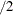in each direction. By default, EXTEND=0.2.

MAXATLEN=length

specifies the maximum number of characters used to display the levels of all the fixed variables. If the text is too long, it is truncated and ellipses ("...") are appended. By default, length is equal to its maximum allowed value, 256.

POLYBAR

replaces scatter plots of polytomous response models with bar charts. This option has no effect on binary-response models, and it is overridden by the CONNECT option.

SHOWOBS<=YES | NO>

displays observations on the plot. For event/trial notation, the observed proportions are displayed; for single-trial binary-response models, the observed events are displayed at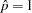and the observed nonevents are displayed at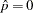. For polytomous response models the predicted probabilities at the observed values of the covariate are computed and displayed.

YRANGE=(<min><,max>)

displays the Y axis as [min,max]. Note that the axis might extend beyond your specified values. By default, the entire Y axis, [0,1], is displayed for the predicted probabilities. This option is useful if your predicted probabilities are all contained in some subset of this range.

### Odds Ratio Plots

When either the CLODDS= option or the ODDSRATIO statement is specified, the resulting odds ratios and confidence limits can be displayed in a graphic. If you have many odds ratios, you can produce multiple graphics, or panels, by displaying subsets of the odds ratios. Odds ratios with duplicate labels are not displayed. See Outputs 51.2.9 and 51.3.3 for examples of odds ratio plots.

The following oddsratio-options modify the default odds ratio plot.

DOTPLOT

displays dotted gridlines on the plot.

GROUP

displays the odds ratios in panels defined by the ODDSRATIO statements. The NPANELPOS= option is ignored when this option is specified.

LOGBASE=2 | E | 10

displays the odds ratio axis on the specified log scale.

NPANELPOS=n

breaks the plot into multiple graphics having at most
odds ratios per graphic. Ifis positive, then the number of odds ratios per graphic is balanced; but ifis negative, then no balancing of the number of odds ratios takes place. By default,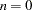and all odds ratios are displayed in a single plot. For example, suppose you want to display 21 odds ratios. Then specifying NPANELPOS=20 displays two plots, the first with 11 odds ratios and the second with 10; but specifying NPANELPOS=-20 displays 20 odds ratios in the first plot and only 1 odds ratio in the second.

ORDER=ASCENDING | DESCENDING

displays the odds ratios in sorted order. By default the odds ratios are displayed in the order in which they appear in the corresponding table.

RANGE=(<min><,max>) | CLIP

specifies the range of the displayed odds ratio axis. The RANGE=CLIP option has the same effect as specifying the minimum odds ratio as min and the maximum odds ratio as max. By default, all odds ratio confidence intervals are displayed.

TYPE=HORIZONTAL | HORIZONTALSTAT | VERTICAL | VERTICALBLOCK

controls the look of the graphic. The default TYPE=HORIZONTAL option places the odds ratio values on the X axis, while the TYPE=HORIZONTALSTAT option also displays the values of the odds ratios and their confidence limits on the right side of the graphic. The TYPE=VERTICAL option places the odds ratio values on the Y axis, while the TYPE=VERTICALBLOCK option (available only with the CLODDS= option) places the odds ratio values on the Y axis and puts boxes around the labels.Previous Page | Next Page | Top of Page